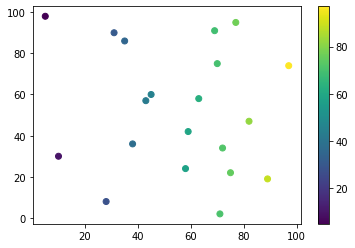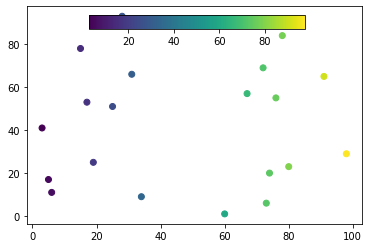# Show Colorbar in Matplotlib

Manav Narula Oct 12, 2021 May 22, 2021 Matplotlib Matplotlib Colorbar

In this tutorial, we will discuss how to show a colorbar in a matplotlib figure in Python.

To create a colorbar we have to use the `matplotlib.pyplot.colorbar()` function.

The following code shows a simple example of this.

``````import random
import matplotlib.pyplot as plt

s_x = random.sample(range(0,100),20)
s_y = random.sample(range(0,100),20)

s = plt.scatter(s_x,s_y,c = s_x, cmap='viridis')

c = plt.colorbar()
``````In the above example, we created a simple colorbar placed outside of the plot. We specified the colormap using the `cmap` parameter.

We can also specify the axes in which we wish to show the colorbar. If we wish, we can add it over the axes of the plot.

For example,

``````import random
import matplotlib.pyplot as plt

s_x = random.sample(range(0,100),20)
s_y = random.sample(range(0,100),20)

fig, ax = plt.subplots()
cax = fig.add_axes([0.27, 0.8, 0.5, 0.05])

im = ax.scatter(s_x,s_y,c = s_x, cmap = "viridis")
fig.colorbar(im, cax = cax, orientation = 'horizontal')
``````In the above example, we used the `subplots()` function to get the figure and axes objects and use it to create the axes for the colorbar. We specified this using the `cax` parameter in the `colorbar()` function.

Also, note the use of the `orientation` parameter which altered the orientation of the final color bar. Apart from this, we can use different parameters to control the shape and size of the colorbar. For example, `shrink` can reduce the size of the colorbar by a small margin, `aspect`, which is the ratio of the sides of the bar, and there are many more.

Author: Manav Narula

Manav is a IT Professional who has a lot of experience as a core developer in many live projects. He is an avid learner who enjoys learning new things and sharing his findings whenever possible.